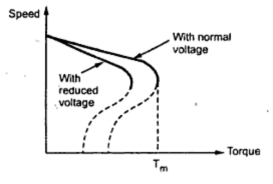### Supply Voltage Control

We know that,  T  α  (k s E22 R2)/(R22 +(s X2)2
Now E2, the rotor induced e.m.f. at standstill depends on the supply voltage V.
...             E2    α   V
Also for low slip region, which is operating region of the induction motor, (s X2)2 <<R2 and hence can be neglected.
...              T  α  ( s E22 R2)/R22α   sV2    for constant R2
Now if supply voltage is reduced below rated value, as per above equation torque produced also decreases. But to supply the same load it is necessary to develope same torque hence value of slip increases so that torque produced remains same. Slip increases means motor reacts by running at lower speed, to decrease in supply voltage. So motor produces the required load torque at a lower speed. The speed-torque characteristics for the motor using supply voltage control are shown in the Fig. 1.Fig.1  Speed-torque curves for motor with voltage control
But in this method, due to reduction in voltage, current drawn by the motor increases. Large change in voltage for small change in speed is required is the biggest disadvantage. Due to increased current, the motor may get overheated. Additional voltage changing equipment is necessary. Hence this method is rarely used in practice. Motors driving fan type of loads use this method of speed control. Due to reduced voltage, E2 decreases, decreasing the value of maximum torque too.